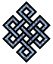fail2ban bad ip database: ip 182.23.64.177

| ip database | live view | stats | report | help | api key:

 ip: 182.23.64.177 hostname: 182.23.64.177 country:[ID] Indonesia first reported: 20.11.2018 20:58.33 GMT+0200 last reported: 06.03.2019 07:02.11 GMT+0200 time period: 105d 10h 03m 38s total reports: 13 reported by: 4 host(s) filter(s): ssh (9) ssh (4) tor exit node no badips.com db Lookupport scan of '182.23.64.177':

[-hide]
```# Nmap 6.40 scan initiated Tue Nov 20 20:59:02 2018 as: /usr/bin/nmap -sU -sS -O 182.23.64.177
Nmap scan report for 182.23.64.177
Host is up (0.17s latency).
Not shown: 1000 open|filtered ports, 994 filtered ports
PORT     STATE  SERVICE
22/tcp   open   ssh
80/tcp   open   http
443/tcp  closed https
2638/tcp closed sybase
8000/tcp closed http-alt
8080/tcp closed http-proxy
No exact OS matches for host (If you know what OS is running on it, see http://nmap.org/submit/ ).
TCP/IP fingerprint:
OS:SCAN(V=6.40%E=4%D=11/20%OT=22%CT=443%CU=%PV=N%G=Y%TM=5BF467B7%P=x86_64-p
OS:c-linux-gnu)SEQ(SP=105%GCD=1%ISR=10A%TI=Z%TS=8)OPS(O1=M5B4ST11NW7%O2=M5B
OS:4ST11NW7%O3=M5B4NNT11NW7%O4=M5B4ST11NW7%O5=M5B4ST11NW7%O6=M5B4ST11)WIN(W
OS:1=7120%W2=7120%W3=7120%W4=7120%W5=7120%W6=7120)ECN(R=Y%DF=Y%TG=40%W=7210
OS:%O=M5B4NNSNW7%CC=Y%Q=)T1(R=Y%DF=Y%TG=40%S=O%A=S+%F=AS%RD=0%Q=)T2(R=N)T3(
OS:R=N)T4(R=N)T5(R=Y%DF=Y%TG=40%W=0%S=Z%A=S+%F=AR%O=%RD=0%Q=)T6(R=N)T7(R=N)
OS:U1(R=N)IE(R=Y%DFI=N%TG=40%CD=S)

OS detection performed. Please report any incorrect results at http://nmap.org/submit/ .
# Nmap done at Tue Nov 20 20:59:51 2018 -- 1 IP address (1 host up) scanned in 50.07 seconds
```
```Σ = 72 | Δt = 0.0038039684295654s
```